Hans Walser, [20090715b]

Regular K-gon and trapezoids

# 1        Example

Starting by a regular yellow heptagon () in the unit circle we add squares on every side. Then we proceed as indicated in the following figure.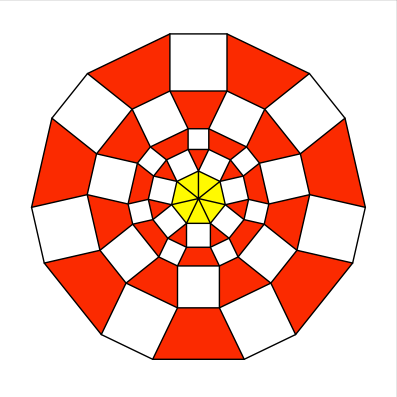Heptagon and squares

We get red isosceles trapezoids between the white squares. In the first ring we see triangles, bat we count them as special trapezoids with upper side zero. We would like to compare the areas of the trapezoids.

# 2        Modification

We can modify the figure by transforming the white squares into rhombuses. This does not change shape or size of the red trapezoids.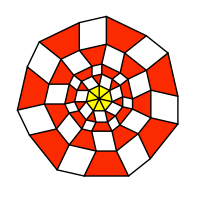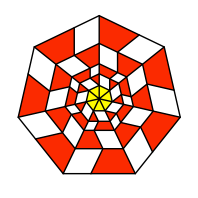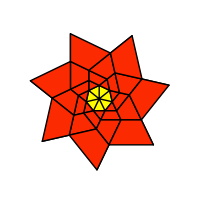Collapsing the squares

# 3        Some calculations

We use the notations of the following figure.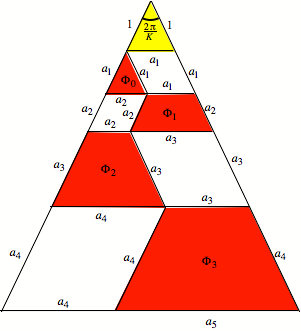Notations

We get:Setting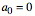we can establish the recursion formula: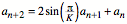For the area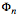we get: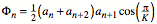Since we want to compare the areas of the red polygons, we introduce the relative area:# 4        Special cases

The tables indicate for different K the numerical values.

## 4.1      K = 1

We get.

## 4.2      K = 2

 n a[n] 0 0 1 2.0 2 4.0 3 10.0 4 24.0 5 58.0 6 140.0 7 338.0 8 816.0

We have “flat” trapezoids. But the squares are interesting.Squares only

If we add only the next square above, we get a rectangle with nearly the shape of the European standard paper shape DIN A.Close to European standard shape DIN A

Indeed we have:n q[n] 0 1.0 1 1.5 2 1.4 3 1.4166667 4 1.4137931 5 1.4142857 6 1.4142012 7 1.4142157 8 1.4142132 9 1.4142136 10 1.4142136

## 4.3      K = 3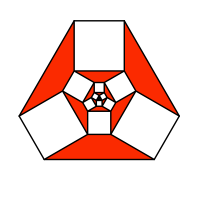K = 3

 n a[n] phi[n] psi[n] 0 0 1.299038106 1.0 1 1.732050808 6.495190528 5.0 2 3.0 31.17691454 24.0 3 6.92820323 149.3893822 115.0 4 15.0 715.7699962 551.0 5 32.90896534 3429.460599 2640.0 6 72.0 16431.533 12649.0 7 157.6166235 78728.20439 60605.0 8 345.0 377209.489 290376.0

We see, thatare integer numbers, but not the. The case

 n a[n] phi[n] psi[n] 1 1.732050808 6.495190528 5.0

is subject of [Deshpande 2009].

We have the recursion formula:## 4.4      K = 4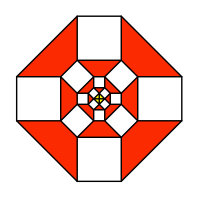K = 4

The Figure on the left fits into a square lattice, but not so the figure on the right.

 n a[n] phi[n] psi[n] 0 0 1.0 1.0 1 1.414213562 4.0 4.0 2 2.0 15.0 15.0 3 4.242640687 56.0 56.0 4 8.0 209.0 209.0 5 15.55634919 780.0 780.0 6 30.0 2911.0 2911.0 7 57.98275606 10864.0 10864.0 8 112.0 40545.0 40545.0

Both theand the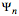are integers. We have the recursion formula: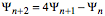## 4.5      K = 5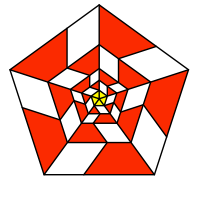K = 5

 n a[n] phi[n] psi[n] 0 0 0.6571638901 1.0 1 1.175570505 2.22250594 3.381966011 2 1.381966011 6.85927566 10.4376941 3 2.800168986 20.9753312 31.91796068 4 4.673762079 64.07858154 97.50776405 5 8.294505831 195.7362536 297.8499832 6 14.42453848 597.8947754 909.8107555 7 25.25156781 1826.323555 2779.099069 8 44.1095368 5578.669413 8489.007836

No integer numbers. Probably there is the golden section in it, but I do not see it. But there is a very interesting recursion formula: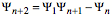## 4.6      K = 6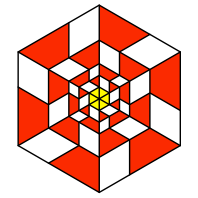K = 6

The figure on the right fits into a regular triangular lattice. The figure on the left not, since squares and regular triangles don’t like each other.

 n a[n] phi[n] psi[n] 0 0 0.4330127019 1.0 1 1.0 1.299038106 3.0 2 1.0 3.464101615 8.0 3 2.0 9.09326674 21.0 4 3.0 23.8156986 55.0 5 5.0 62.35382907 144.0 6 8.0 163.2457886 377.0 7 13.0 427.3835368 987.0 8 21.0 1118.904822 2584.0

Theare the Fibonacci numbers (), and theare every second Fibonacci number ().We have the recursion formula:## 4.7      K = 7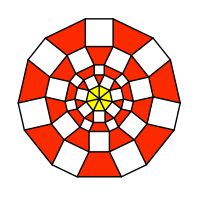K  = 7

 n a[n] phi[n] psi[n] 0 0 0.2943675264 1.0 1 0.8677674782 0.8103998041 2.753020396 2 0.7530203963 1.936679664 6.579121302 3 1.521214089 4.521318811 15.35943474 4 2.07308051 10.51060324 35.70571581 5 3.320165935 24.41458629 82.93912915 6 4.95421253 56.70325077 192.6273984 7 7.619270449 131.6906196 447.3680275 8 11.56596763 305.8437111 1038.985906

Awful numbers. We have again the interesting recursion formula:## 4.8      K = 8K = 8

 n a[n] phi[n] psi[n] 0 0 0.2071067812 1.0 1 0.7653668647 0.5355339059 2.585786438 2 0.5857864376 1.17766953 5.686291501 3 1.213708394 2.509667992 12.11774901 4 1.514718626 5.311795927 25.64761953 5 2.373023839 11.22550187 54.20151774 6 3.330952442 23.71495458 114.5059299 7 4.922424466 50.09630604 241.8863629 8 7.098413022 105.8233942 510.9605468

We have again the interesting recursion formula:# 5        Summary

Cases with integer numbers only for K = 2, 3, 4, 6.

In the general case there is the conjecture:If anybody has time to prove it, I would be glad to hear about.

References

[Deshpande 2009]      Deshpande, M. N. : Proof Without Words: Beyond Extriangles. MATHEMATICS MAGAZINE. Vol. 82, No. 3, June 2009, p. 208.